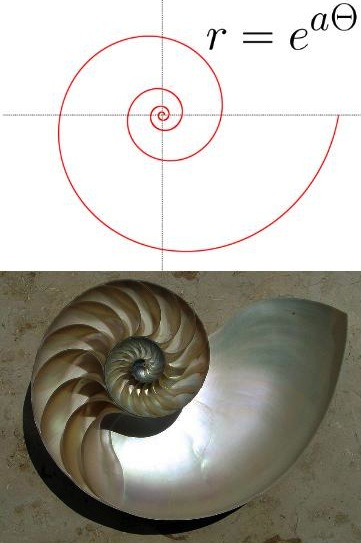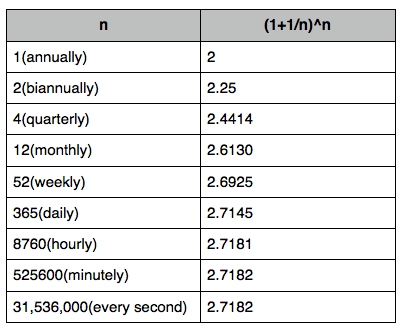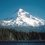# This note has been used to help create the The Discovery of the Number e wikie Logarithmic Spiral

The more math and science you encounter, the more you run into the number $e$. Many of its applications and manifestations are alarmingly beautiful and often abstract. The logarithmic spiral is a shape that appears in nature, commonly in: shells, horns, tusks, sunflowers, and spiral galaxies. Despite the aesthetic wonders of the number e, it was actually first discovered in a pragmatic financial investigation of the behavior of compound interest.

When most of us are first taught about the number $e$, we are told that it is an irrational, transcendental number whose value is approximated by $2.7182$. Most people simply learn to manipulate $e$. High school classes rarely mention where $e$ comes from. It is usually introduced when learning about exponents and logarithms as a “special” base that you will use a lot down the road. Early in high school I remember asking a teacher what $e$ was. I received the usual circular answer, that $e$ is the base of the natural logarithm which in turn is the logarithm of an exponent raised to the base of $e$. This answer did not satisfy me, but I was told that I had to wait for calculus to learn other ways of approaching its definition.

$e$ makes profound appearances in calculus. That said, $e$ was first discovered with algebra in the 17th century. Mathematicians indirectly came close to $e$ many times without directly calculating or recognizing it as anything out of the ordinary. $e$ was almost discovered when logarithms were invented in 1618 by John Napier. Logarithms immensely aided longhand computation by allowing multiplication and division to be carried out by addition and subtraction. These logarithms were not the same as our current conception of a logarithm. They were numbers that aided computation, and were not yet thought of as functions that relate exponents to their bases. Mathematicians effectively computed tables of the natural logarithm but did not know that the number e was anywhere behind them. Historians are not sure who exactly was the first person to calculate $e$ and recognize it as special. Most likely, $e$ was not discovered by a mathematician, but by someone with more worldly motivations. The number $e$ lies at the foundations of one of the most fundamental processes of finance: compound interest.

The 17th century was a time of rapid change. It was the era of the Scientific Revolution, the proliferation of colonialism, the emergence of mass literacy, and an explosion of international trade.The European Age of Exploration (and exploitation) brought disparate cultures of the world in contact, conflict, and business with each other to a degree that none of the large empires of old ever approached. Increasing the scale of commerce increased the demands for capital. Money lending began to play an increasingly large role in the prosperity of individuals, businesses ventures, and nations.

Given the growing presence of finance in the 17th century, historians believe that the first person to calculate $e$, was most likely a banker or trader exploring the properties of compound interest.

Interest is a fee charged by a lender on a borrower, for the service of providing a loan. Interest fees offset the opportunity cost to the lender of not being able to do anything else with their assets while they are controlled by the borrower. These interest fees accumulate over time, depending on the interest rate. Borrowers think of interest as the cost of having debt, while lenders think of interest as the return on their loan as an investment.

Simple interest is where the interest accumulated is always the same proportion of the initial amount borrowed or invested(this initial amount is called the principal). For instance, if you borrow $1.00 at 5% interest per year, after one year, you will owe$1.05. After two years you will owe $1.10, etc. In effect, simple interest creates an arithmetic progression where a debt or investment grows at the same rate over all periods of time. Simple interest is rare and usually appears only in short term loans. Compound interest is where interest accumulates on both the principal and the prior interest. Each time the interest rate is applied to the total accumulated debt or investment, it is referred to as compounding. If you invest$1 at 5% annual compounding interest, after the first year you would have $1.05. Unlike simple interest, in the second year it would increase by 5% of$1.05, yielding $1.1025. The general equation for compound interest, compounded once annually is $S=P(1+r)^{t},$ where $S$ is the total accumulated debt or investment, $P$ is the principal, $r$ is the interest rate and $t$ is the time elapsed in years. Under compound interest, debts and investments grow by a geometric progression. Interest can be compounded multiple times within a given year or interest rate period. For instance, if you put one dollar into a bank account that returns 5% interest per year compounded biannually, then your account will grow by 2.5% twice in a given year. For multiple compounding intervals within each rate period, the formula for compound interest becomes: $S=P \left( 1+\frac{r}{n} \right)^{nt}$ where $n$ is the number of times the interest is compounded in a given year and $nt$ is therefore the total number of times it is compounded.Compound Interest Table Compounding more times in a given time period causes your debt or investment to grow more often, but at a smaller rate each time it is compounded. As you can see, it would not take much imagination for an ambitious banker to wonder how much money could be made if the interest rate was really high and it was compounded as much as possible (daily, hourly, infinitely, etc.). If more compounding intervals make investments grow more often, would more compounding result in investments growing faster? Historians believe that a businessman or banker likely beat the mathematicians to the thought experiment: if$1 is invested at 100% interest over one year, how much more money will be made if it is compounded often as opposed to only compounding it a few times in a year??

The equation for compound interest in this special case would simplify to:

$S= \left(1+\frac{1}{n} \right)^{n}.$

It turns out that compounding weekly barely yields any more money than compounding monthly and at higher values of $n$, it gets closer and closer to what we recognize as the number $e$. As you can see, compounding more often does yield more money up to a point, but rapidly reaches an upper bound where growing more often, does not yield faster growth.Mathematicians would later go on to define $e$ as the limit:

$\lim_{n \to \infty} \left( 1+1/n \right)^{n}=e.$

With $n$ going to infinity, growth occurs continuously, at every possible instant no matter how small the time interval. Simple interest reflects an arithmetic growth progression, compound interest reflects a discrete geometric growth progression, and infinitely compounding interest reflects a continuous growth progression(exponential growth). The number $e$ is thought of as the base that represents the growth of processes or quantities that grow continuously in proportion to their current quantity. This is why e appears so often in modeling the exponential growth or decay of everything from bacteria to radioactivity.

Join the conversation at the Brilliant.org discussions page.

For a wordier and more visual explanation of $e$, check out this this piece.

For a more detailed account of the history of 17th century mathematics surrounding the emergence of $e$, check out this page.

For most everything you could ever want to know about $e$, read:

e: the Story of a Number, by Eli Maor - This a history book written for a general audience. There is some calculus in it, in the best of ways. It is very well explained and introduced, anybody with comfort or interest in basic math would embrace his story telling.Note by Peter Taylor
7 years, 3 months ago

This discussion board is a place to discuss our Daily Challenges and the math and science related to those challenges. Explanations are more than just a solution — they should explain the steps and thinking strategies that you used to obtain the solution. Comments should further the discussion of math and science.

When posting on Brilliant:

• Use the emojis to react to an explanation, whether you're congratulating a job well done , or just really confused .
• Ask specific questions about the challenge or the steps in somebody's explanation. Well-posed questions can add a lot to the discussion, but posting "I don't understand!" doesn't help anyone.
• Try to contribute something new to the discussion, whether it is an extension, generalization or other idea related to the challenge.

MarkdownAppears as
*italics* or _italics_ italics
**bold** or __bold__ bold
- bulleted- list
• bulleted
• list
1. numbered2. list
1. numbered
2. list
Note: you must add a full line of space before and after lists for them to show up correctly
paragraph 1paragraph 2

paragraph 1

paragraph 2

[example link](https://brilliant.org)example link
> This is a quote
This is a quote
    # I indented these lines
# 4 spaces, and now they show
# up as a code block.

print "hello world"
# I indented these lines
# 4 spaces, and now they show
# up as a code block.

print "hello world"
MathAppears as
Remember to wrap math in $$ ... $$ or $ ... $ to ensure proper formatting.
2 \times 3 $2 \times 3$
2^{34} $2^{34}$
a_{i-1} $a_{i-1}$
\frac{2}{3} $\frac{2}{3}$
\sqrt{2} $\sqrt{2}$
\sum_{i=1}^3 $\sum_{i=1}^3$
\sin \theta $\sin \theta$
\boxed{123} $\boxed{123}$

Sort by:

Well stated and written!

- 7 years, 1 month ago

interesting!

- 7 years ago

GREAT! I LIKED. I HAD NO IDEA !

- 6 years, 10 months ago

Well written

- 7 years, 1 month ago

This was something I wanted to write. Especially the banking part.

- 7 years, 1 month ago